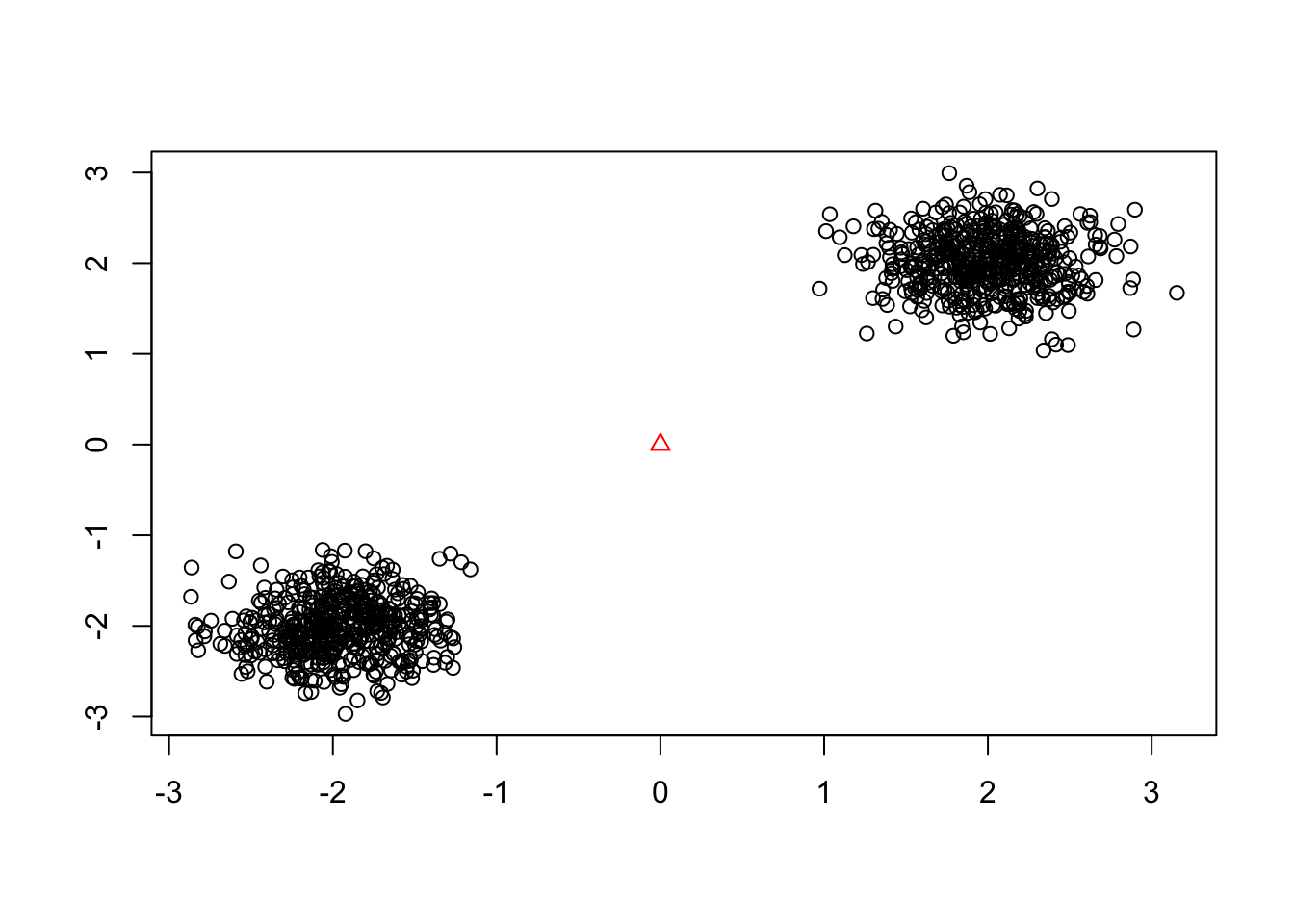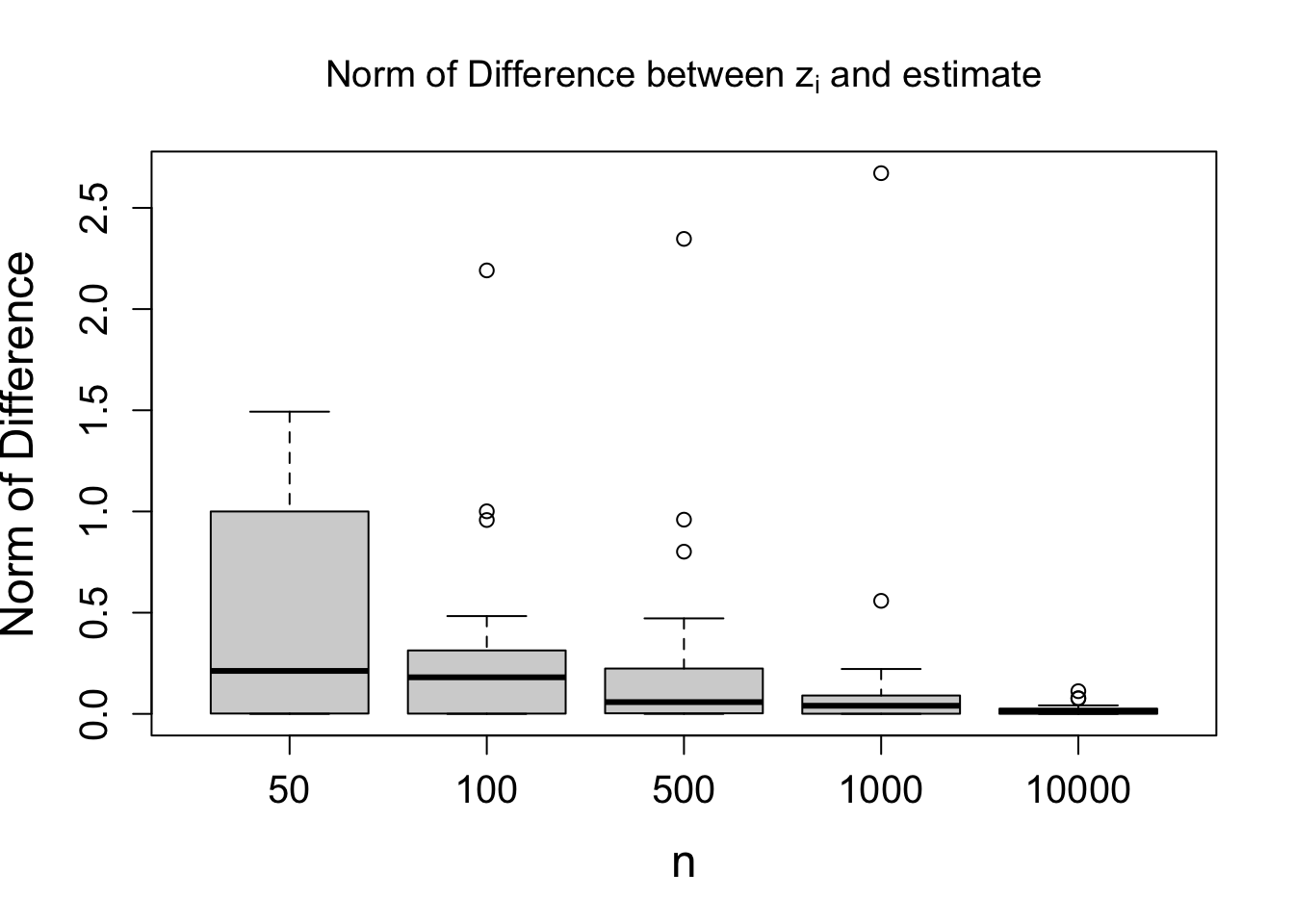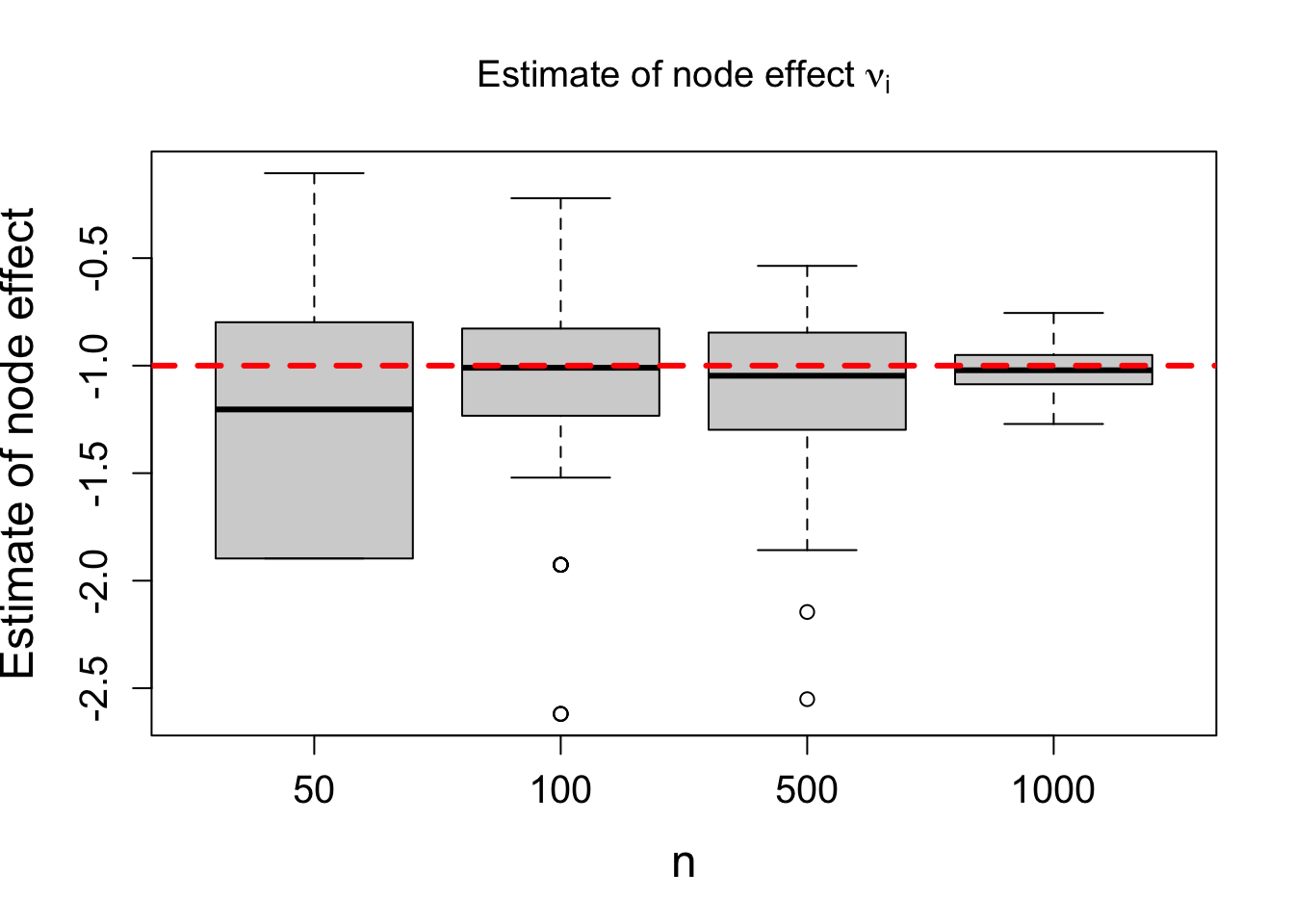## Introduction

This R Markdown file illustrates the estimation procedure for the node location and effects described in Theorem 1 of our paper Consistently estimating network statistics using aggregated relational data." Our paper aims to use Aggregated Relational Data (ARD) to estimate network parameters of a common network model. ARD is an easy-to-collect alternative to complete network data that asks questions of the form “How many people with trait $$X$$ do you know?” for a set of traits that the researcher selects. See our paper for a list of references and applications of ARD.

## Problem Setup

We now formally describe the problem. We assume the following generative model for the unobserved network $$g$$ of interest. Each node $$i \in \{1, \dotsc, n\}$$ has a latent space location $$z_i^\star \in \mathcal{M}$$, where $$\mathcal{M}$$ is either a Euclidean, spherical, or hyperbolic space, as well as a node effect $$\nu_i^\star$$. Nodes are assigned to one of $$K$$ groups, and given an assignment to group $$k$$, node $$i's$$ location on $$\mathcal{M}$$ is drawn from some distribution $$F(\mu_k, \sigma_k)$$. In the Euclidean case, $$F$$ might be a $$p$$-dimensional Gaussian distribution with mean $$\mu_k \in \mathbb{R}^p$$ and covariance matrix $$\sigma_k^2 I_p$$. Conditioned on these variables, edges in this undirected network form independently with probability $\begin{equation} \label{eq: main_model} P(g_{ij} = 1 \mid \nu_i^\star, \nu_j^\star, z_i^\star, z_j^\star) = \exp\{\nu_i^\star + \nu_j^\star - d_{\mathcal{M}}(z_i^\star, z_j^\star)\} \end{equation}$ where $$d_{\mathcal{M}}(z_i^\star, z_j^\star)$$ is the distance between $$z_i^\star$$ and $$z_j^\star$$ along the surface of the latent space $$\mathcal{M}$$.

Our goal in this work is to estimate the locations and node effects of all nodes in the network. However, we do not observe $$g$$ directly. Instead, we only have access to ARD responses. Specifically, for a collection of $$m << n$$ nodes, we have access to $$Y_{ik} := \sum_{j \in G_k} g_{ij}$$ for $$i = 1, \dotsc, m$$ and $$k = 1, \dotsc, K$$, where $$G_k$$ is the set of nodes in group $$k$$ and $$g = \{g_{ij}\}$$ is the unobserved network of interest. In other words, we survey $$m$$ distinct nodes in the network, and ask each of them how many people they know with trait $$k$$ for all traits $$k = 1, \dotsc, K$$. One contribution in this work is to estimate the locations and node effects of nodes in our survey. Since we do not collect data from nodes not in our survey, it is not possible to estimate the parameters for these nodes not in our ARD survey.

The main insight behind our estimation procedure is to recognize that the ARD responses are of the form $$Y_{ik} = \sum_{j \in G_k} g_{ij}$$ where $$G_k$$ is the set of nodes in group $$k$$ and $$g = \{g_{ij}\}$$ is the unobserved network of interest. At a high level, to estimate the node locations and node effects, we equate the observed data $$Y_{ik}$$ with the probability that node $$i$$ connects to other nodes in the network. This probability, which we denote by $$p_{ik}$$, depends on the unknown location and node effect of node $$i$$ - thus we write $$p_{ik} = p_{ik}(z_i^\star, \nu_i^\star)$$. More correctly, this probability also depends on the unknown model parameters $$(\mu_1, \dotsc, \mu_K, \sigma_1, \dotsc, \sigma_K, E(\exp(\nu)))$$, but for simplicitiy we assume these are known. Our paper also proposes consistent estimators for these model parameters using similar method-of-moments estimators.

# Simulation Setup

We simulate two group centers $$\mu_1 = (2, 2)$$ and $$\mu_2 = (-2, -2)$$. For each group, we simulate $$n_k$$ locations drawn independently from $$N(\mu_k, \frac{1}{3} I_2)$$. Our goal is to estimate the location of a reference node $$z_0 = (0, 0)$$.

Below we plot the simulation results for $$n = 500$$. In black we plot the locations of nodes in group 1 and 2, and the red triangle at (0, 0) denotes the location of the reference node.

n_k = 500
group_1_location = matrix(rnorm(2 * n_k, 2, 1/3), ncol = 2)
group_2_location = matrix(rnorm(2 * n_k, -2, 1/3), ncol = 2)
plot(rbind(group_1_location, group_2_location), xlab = "", ylab = "")
points(0, 0, pch = 2, cex = 1, col = "red")We now simulate the ARD responses $$Y_{i1}$$ and $$Y_{i2}$$, by $\begin{equation*} Y_{i1} = \sum_{j \in G_1} g_{ij}, \ \ \ Y_{i2} = \sum_{j\in G_2} g_{ij} \end{equation*}$ where $$g_{ij} \sim \text{Bernoulli}(p_{ij})$$ and $$p_{ij} = \exp(-||z_0 - z_j||)$$. Note to simplify the exposition we assume $$\nu_j^\star = 0$$ in the simulations for the node location estimator. In the simulations for the node effects later on, we take them to be non-zero.

The code below uses a handy trick for quickly computing the distance between one point and a vector of points.

z_0 = c(0, 0)
dist_1 = apply(t(group_1_location), 2, function(point) {norm(z_0 - point, "2")})
dist_2 = apply(t(group_2_location), 2, function(point) {norm(z_0 - point, "2")})
P_group_1 = exp(-dist_1)
P_group_2 = exp(-dist_2)
Y_i1 = 0
Y_i2 = 0
while(sum(Y_i1) == 0 || sum(Y_i2) == 0){
Y_i1 = rbinom(n_k, 1, P_group_1)
Y_i2 = rbinom(n_k, 1, P_group_2)
}

## Performance of node location estimate.

Given the ARD responses $$Y_{i1}$$ and $$Y_{i2}$$, our goal is to estimate the location of the reference node $$z_0 = (0, 0)$$. See the main paper for a complete discussion of our estimator, but we provide the intuition and quick description of the estimator below. Since $$Y_{ik} | z_i^\star$$ has a Binomial distribution, by the weak law of large numbers, $$Y_{ik}/n_k$$ converges in probability to $$p_{ik}$$, the probability that node $$i$$ connects to an arbitrary node in group $$k$$. This probability depends on the unknown latent space location $$z_i$$ and the node effect of node $$i$$. In the case where $$\nu_j^\star = 0$$ for all nodes, we have that $\begin{equation*} p_{ik} = \int_{\mathcal{M}} \exp(-d(z_i, z)) \ f_k(z) dz \end{equation*}$ where $$f_k$$ is the distribution of nodes in group $$k$$. By equating $$Y_{ik}/n_k$$ with $$p_{ik}$$ for several values of $$k$$, we can estimate the location of node $$i$$. For example, using two groups, we solve the following system of equations for $$z_i^\star$$. $\begin{equation*} \begin{pmatrix} Y_{i1} n_1^{-1} \\ Y_{i2} n_2^{-1} \\ \end{pmatrix} = \begin{pmatrix} p_{i1}(z_i^\star)\\ p_{i2}(z_i^\star) \end{pmatrix} \end{equation*}$ Below we plot the norm $$||z_i - \hat z_i|| = ||\hat z_i||$$ for various values of $$n$$. We see that as $$n$$ increases, the norm is decreasing, which shows that our estimate is getting closer to the true location.

library(latex2exp)
n.vec = c(50, 100, 500, 1000, 10^4) # n.vec is the sample size used to compute the estimate of z_i*
diff = readRDS("diff_z.rds") # load in the saved values of the norm difference.
boxplot(diff, names = n.vec, cex.lab = 1.5, cex.axis = 1.25, xlab = "n", ylab = "Norm of Difference", main=TeX('Norm of Difference between $\\z_i$ and estimate'))## Results for node effect estimation

Our estimate of the node location is, generally speaking, a method-of-moments estimate. We follow a similar approach to derive our estimate of the node effects. Using the generative model for the network, we know that $$Y_{ik} | z_i^\star, \nu_i^\star \sim \text{Binomial}(n_k, p_{ik})$$ where $\begin{equation*} p_{ik} = E\{\exp(\nu_i^\star)\} \exp(\nu_i) \int_{\mathcal{M}} \exp(-d(z_i, z)) \ f_k(z) dz \;. \end{equation*}$ Assuming we know $$E\{\exp(\nu_i^\star)\}$$ (and again, our paper proposes consistent estimates of this parameter) and can approximate the term $$\int_{\mathcal{M}} \exp(-d(z_i, z)) f_k(z) dz$$, we can equate this equation with $$Y_{ik}/n_k$$ and solve for $$\nu_i^\star$$. We can approximate this integral by plugging in the estimated value $$\hat z_i$$.

Below we plot the results of this estimation procedure for various values of $$n_k = n$$. The true value of $$\nu_i^\star = -1$$. As $$n$$ increases, we see that the estimate is convering to the true value.

library(latex2exp)
n.vec = c(50, 100, 500, 1000) # n.vec is the sample size used to compute the estimate of nu_i*
xlab = "n", ylab = "Estimate of node effect", main=TeX('Estimate of node effect $\\nu_i$'))
abline(h =-1, col="red", lwd=3, lty=2)